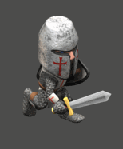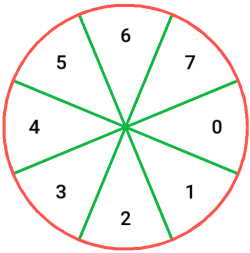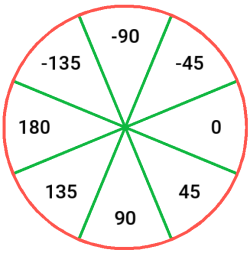# 8-Directional Movement/Animation

## Problem

You need a 2D character that has 8-directional movement, including animation.

## Solution

For our example, we’ll use the Isometric Mini-Crusader, which contains 8-directional animations for idle, run, attack, and several other states.The animations are organized in folders, with a separate image for each frame. We’ll use an `AnimatedSprite` and we’ll name each animation based on its direction. For example, `idle0` pointing to the right and going clockwise to `idle7`.

When our character moves, it will pick an animation based on the direction of movement:We’ll use the mouse to move - the character will always face the mouse and run in that direction when we click the mouse button.

To choose which animation to play, we need to get the mouse direction and map it to this same range of `0-7`. `get_local_mouse_position().angle()` gives us the angle to the mouse (in radians). Using `stepify()` to snap this to the closest multiple of 45° (`PI/4` radians) gives the following result:Divide each by 45° (`PI/4` radians), and we have:Finally, we need to map the resulting range to `0-7` using the modulus (`%`) operator, and we’ll have our correct values. Adding that value to the end of the animation name (“idle”, “run”, etc) gives us the correct animation:

``````func _process(delta):
current_animation = "idle"

var mouse = get_local_mouse_position()
a = stepify(mouse.angle(), PI/4) / (PI/4)
a = wrapi(int(a), 0, 8)

if Input.is_action_pressed("left_mouse") and mouse.length() > 10:
current_animation = "run"
move_and_slide(mouse.normalized() * speed)

\$AnimatedSprite.animation = current_animation + str(a)
``````### Keyboard input

If you’re using keyboard controls instead of mouse, you can get the angle of movement based on which keys are being held. The rest works in the same way.

``````func _process(delta):
current_animation = "idle"
var input_dir = Vector2.ZERO
if Input.is_action_pressed("right"):
input_dir.x += 1
if Input.is_action_pressed("left"):
input_dir.x -= 1
if Input.is_action_pressed("down"):
input_dir.y += 1
if Input.is_action_pressed("up"):
input_dir.y -= 1
input_dir = input_dir.normalized()
if input_dir.length() != 0:
a = input_dir.angle() / (PI/4)
a = wrapi(int(a), 0, 8)
current_animation = "run"
move_and_slide(input_dir * speed)

\$AnimatedSprite.animation = current_animation + str(a)
``````